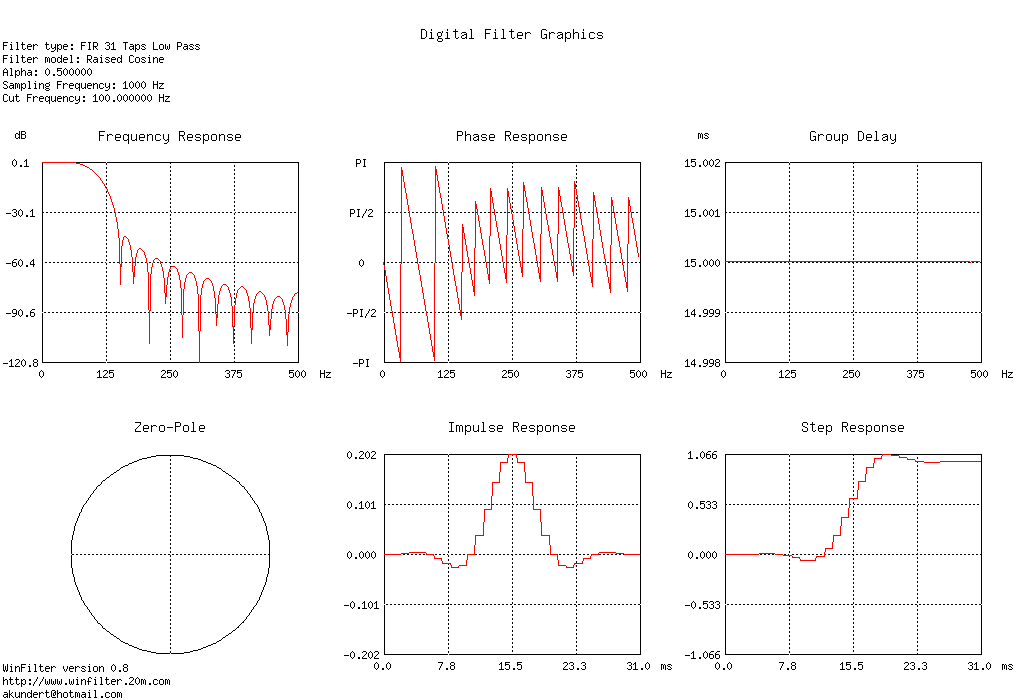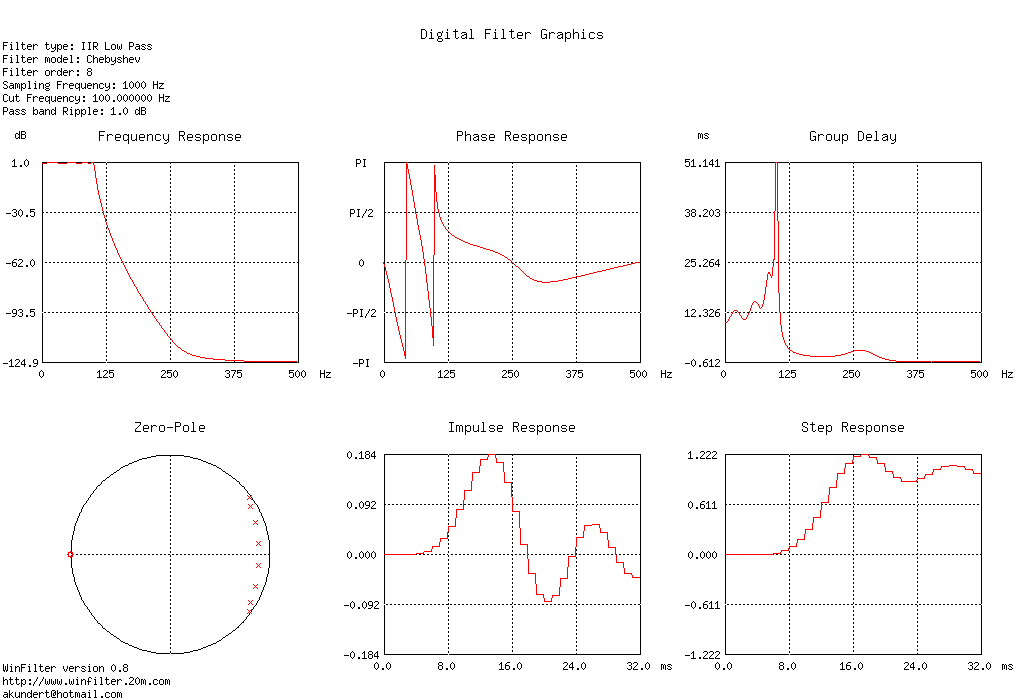http://www.winfilter.20m.com

This software can design as well IIR filters as FIR filters and can generate the C and VHDL code.

Generierung von VHDL-Code für IIR-Filter nicht implementiert.Generierter C-Code:
fir.c
/**************************************************************
WinFilter version 0.8
http://www.winfilter.20m.com
akundert@hotmail.com

Filter type: Low Pass
Filter model: Raised Cosine
Roll Off Factor: 0.500000
Sampling Frequency: 1000 Hz
Cut Frequency: 100.000000 Hz
Coefficents Quantization: 16-bit
***************************************************************/

#define Ntap 31

#define DCgain 131072

__int16 fir(__int16 NewSample) {
__int16 FIRCoef[Ntap] = {
0,
69,
302,
573,
602,
85,
-1068,
-2531,
-3521,
-3019,
-201,
5093,
12099,
19226,
24557,
26533,
24557,
19226,
12099,
5093,
-201,
-3019,
-3521,
-2531,
-1068,
85,
602,
573,
302,
69,
0
};

static __int16 x[Ntap]; //input samples
__int32 y=0;            //output sample
int n;

//shift the old samples
for(n=Ntap-1; n>0; n--)
x[n] = x[n-1];

//Calculate the new output
x = NewSample;
for(n=0; n<Ntap; n++)
y += FIRCoef[n] * x[n];

return y / DCgain;
}Generierter C-Code:
iir.c
/**************************************************************
WinFilter version 0.8
http://www.winfilter.20m.com
akundert@hotmail.com

Filter type: Low Pass
Filter model: Chebyshev
Filter order: 8
Sampling Frequency: 1000 Hz
Cut Frequency: 100.000000 Hz
Pass band Ripple: 1.000000 dB
Coefficents Quantization: float

Z domain Zeros
z = -1.000000 + j 0.000000
z = -1.000000 + j 0.000000
z = -1.000000 + j 0.000000
z = -1.000000 + j 0.000000
z = -1.000000 + j 0.000000
z = -1.000000 + j 0.000000
z = -1.000000 + j 0.000000
z = -1.000000 + j 0.000000

Z domain Poles
z = 0.850893 + j -0.323757
z = 0.850893 + j 0.323757
z = 0.884821 + j -0.114819
z = 0.884821 + j 0.114819
z = 0.809355 + j -0.481042
z = 0.809355 + j 0.481042
z = 0.793689 + j -0.574205
z = 0.793689 + j 0.574205
***************************************************************/

#define NCoef 8
float iir(float NewSample) {
float ACoef[NCoef+1] = {
0.00000125614733130704,
0.00001004917865045632,
0.00003517212527659711,
0.00007034425055319422,
0.00008793031319149277,
0.00007034425055319422,
0.00003517212527659711,
0.00001004917865045632,
0.00000125614733130704
};

float BCoef[NCoef+1] = {
1.00000000000000000000,
-6.67751678724365230000,
20.18180456038727400000,
-35.97553530381035400000,
41.30682183420727700000,
-31.25387137874673700000,
15.21102606778128300000,
-4.35370698763383320000,
0.56131294913559848000
};

static float y[NCoef+1]; //output samples
static float x[NCoef+1]; //input samples
int n;

//shift the old samples
for(n=NCoef; n>0; n--) {
x[n] = x[n-1];
y[n] = y[n-1];
}

//Calculate the new output
x = NewSample;
y = ACoef * x;
for(n=1; n<=NCoef; n++)
y += ACoef[n] * x[n] - BCoef[n] * y[n];

return y;
}

Siehe auch